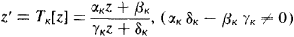# Automorphic Function

The following article is from The Great Soviet Encyclopedia (1979). It might be outdated or ideologically biased.

## Automorphic Function

in mathematics, an analytic function whose values do not change if its argument is subjected to certain linear fractional transformations. Automorphic functions include periodic functions and, in particular, elliptic functions.

For example, if the transformations are integer-valued and have the form of z’ = z + ω, where ω is a nonzero complex number, we obtain automorphic functions characterized by the equation f (z + ω) = f (z)—that is, periodic functions with period ω. In this case the transformation which does not modify the function is a shift of the plane by the vector ω. Obviously, the same shift repeated an arbitrary number of times will likewise not change the function. As a result we obtain a group of linear transformations z’ = z + n ω (n = 0, ±1, ±2, . . . ) which do not modify f (z). In the general case, assume that Γ is a group of linear fractional transformationsand that G is a region which is mapped onto itself by each of these transformations. Then a function f which is single-valued and analytic in G is an automorphic function, with respect to the group Γ, if f[TK (z)] = f (z) for k= 1, 2, . . . . The most important case is the one in which G is a circle or half-plane. Such a region may be regarded as the image of a Lobachevskii plane, and the transformations of Γ may be treated as motions in that plane. The corresponding automorphic functions may be regarded as such a generalization of periodic functions when shifts in a Euclidean plane are replaced by motions in a Lobachevskii plane. This point of view, developed by Poincaré, was the cornerstone of the successful construction of a general theory of automorphic functions. (Prior to Poincaré, important results in this field had been achieved by F. Klein.) In general, the entire theory of automorphic functions at present is a remarkable example of the fruitfulness of Lobachevskii’s geometrical concepts as applied to mathematical analysis and the theory of functions.

Besides problems of conformal mapping, other approaches to general automorphic functions are the theory of linear differential equations, the study of algebraic curves of order higher than the fourth, the solution of algebraic equations (for instance, the solution of general fifth-degree equations with one unknown involves automorphic functions), and others.

### REFERENCES

Ford, L. R. Avtomorfnye funktsii. Moscow-Leningrad, 1936. (Translated from English.)
Klein, F. Lektsii o razvitii matematiki v 19 stoletii, part 1. Moscow-Leningrad, 1937. Chapter 8. (Translated from German.)
Golubev, V. V. Lektsii po analiticheskoi teorii differentsial’nykh uravnenii, 2nd ed. Moscow-Leningrad, 1950.
Golubev, V. V. Odnoznachnye analiticheskie funktsii: Avtomorfnye funktsii. Moscow, 1961.
References in periodicals archive ?
A is a linear densely defined sectorial operator on a complex Banach space X, F : R x X x X [right arrow] X, and (t, x, y) [right arrow] F(t, x, y) is a weighted pseudo almost automorphic function in te R for each x, y [member of] X satisfying suitable conditions.
for short, iff there exist a function h [member of] [C.sub.0]([0, [infinity]) : X) and a (compact) almost automorphic function q : R [right arrow] X such that f(t) = h(t) + q(t), t [right arrow] 0.
Let X and Y be two Banach spaces and f : [bar.[q.sup.Z]] [right arrow] X an almost automorphic function. If [phi] : X [right arrow] Y is a continuous function, then the composite function [phi](f) : [bar.[q.sup.Z]] [right arrow] Y is almost automorphic.
The range of an almost automorphic function is relatively compact on X and hence is bounded.
Moreover, Diagana also introduced Stepanov-like almost automorphic functions which are a generalization of the classical almost automorphic functions; for more details, see .
The real motivation of considering such magnetic quantum Hamiltonian, and therefore the corresponding magnetic potential vector in (38), lies in the study of the space of biweighted holomorphic automorphic functions (defined by the means of the projective representation discussed in Section 4).
The space of almost automorphic functions (resp., compact almost automorphic functions) will be written as AA(X) (resp., [AA.sub.c](X)).
Since the space W P AP(T x X, X) is a particular case of the space W P AA(T x X, X) (i.e., the weighted pseudo almost periodic functions on time scales are a particular case of the weighted pseudo almost automorphic functions on time scales) and some of the results in [U.sub.B] are also true for [U.sub.[infinity]], Theorems 4.15-4.18 can be deduced directly from Ref.
They cover scattering theory, automorphic functions, field theory, the theory of solitons, quantum groups, and knots.
Notice that it is also possible to consider E = PAP(R, C), AA(R, C), AAA(R, C) or PAA(R, C), namely, the sets of pseudo-almost periodic functions, almost automorphic functions, asymptotically almost automorphic functions and pseudo-almost automorphic functions respectively, see for instance [4,5,9,10,24, 27, 30, 33].
The major themes addressed are hyperbolic geometry, automorphic functions, and applications to number theory; ordinary differential equations and dynamical systems; celestial mechanics and related problems; functions of several complex variables; algebraic topology and the Poincare conjecture; partial differential equations of mathematical physics; probability; continuous groups (Lie groups) and their algebras; the principle of relativity and the Poincare group; applied physics; and the philosophy of science.
BURNSIDE, On a class of automorphic functions, Proc.

Site: Follow: Share:
Open / Close